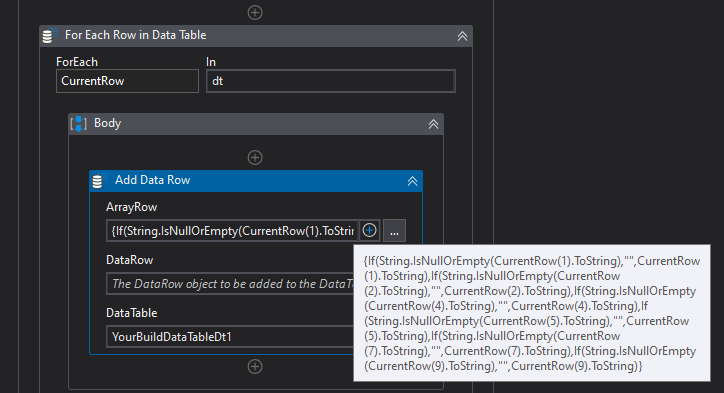# For each excel row, get the cell after "A" and put it into the datable of Column A

Hi guys,

I want to see if it is possible to get data from the cell after A to column of A in uipath data table, and cell after B in to column of B in uipath datatable and so on and so forth.

from this:

to something like this:

Hi @Ben_siu

Do you have column headers for those in the excel file?
if no you can try this

``````{CurrentRow(1).ToString,CurrentRow(2).ToString,CurrentRow(4).ToString,CurrentRow(5).ToString,CurrentRow(7).ToString,CurrentRow(9).ToString}
``````

if yes you can try like this
Same as above instead of numbers inbetween you need to use the column name like

``````{CurrentRow("Column Name 1").ToString,CurrentRow("Column Name 2").ToString,CurrentRow("Column Name 3").ToString,CurrentRow("Column Name 4").ToString,CurrentRow("Column Name 5").ToString,CurrentRow("Column Name 6").ToString}
``````

You can use your build datatable after populating the Datatable.

Hope this Helps

Regards
Sudharsan

1 Like

hi,
thank you this worked for me. But can I make this more inclusive on a larger scale?
For example, I have some rows of data that doesnt have input after A, and I want it to add empty cells to the datatable.

For example:

sometimes there’s no input after the cells(highlighted), So Its hard for me to specify the row positions at all given time, is there a way to insert an empty cell after cells like this?

hi,
so this essentially removes all the Columns with “A” and “B” and “C” right?
But I also want to save the data under the header of “A”/“B”/“C”, can I achieve that by the filter data table activity too?

Okay Check with this expression @Ben_siu

``````{If(String.IsNullOrEmpty(CurrentRow(1).ToString),"",CurrentRow(1).ToString),If(String.IsNullOrEmpty(CurrentRow(2).ToString),"",CurrentRow(2).ToString),If(String.IsNullOrEmpty(CurrentRow(4).ToString),"",CurrentRow(4).ToString),If(String.IsNullOrEmpty(CurrentRow(5).ToString),"",CurrentRow(5).ToString),If(String.IsNullOrEmpty(CurrentRow(7).ToString),"",CurrentRow(7).ToString),If(String.IsNullOrEmpty(CurrentRow(9).ToString),"",CurrentRow(9).ToString)}
``````Same as that if you have column name use “Column Name” instead of numbers

Hope this Helps

Regards
Sudharsan

1 Like

hi, i am putting this “add data row” activity under “for each excel row” because the original data input in from excel, is this correct? And using the input you provided me, it gave me an error message: Option strict on disallows conversion from integer to string.

what have I done wrong in this step?

No add data row should be inside the for each row @Ben_siu

1 Like

Thanks a lot! This works like wonders!!!

This is mostly what I need right now, thank you very much. Also, I would like to know if it is possible to process up to 20 columns of cells which might include empty and null cells.

,If(String.IsNullOrEmpty(CurrentRow(13).ToString),“”,CurrentRow(13).ToString),If(String.IsNullOrEmpty(CurrentRow(14).ToString),“”,CurrentRow(14).ToString),If(String.IsNullOrEmpty(CurrentRow(15).ToString),“”,CurrentRow(15).ToString),If(String.IsNullOrEmpty(CurrentRow(16).ToString),“”,CurrentRow(16).ToString),If(String.IsNullOrEmpty(CurrentRow(17).ToString),“”,CurrentRow(17).ToString)

according to your function I modified it to something like this, but and error message poped up “cannot find column 13”, I guess its because my experimental data didn’t fill in “,” in column 13 and after. so the cell is not recognise as empty, is there a way to tackle this?

Yes that may be the reason check if you have column 13 in your dt @Ben_siu

1 Like

Not all rows have 13 or more data columns, is it possible to modify my workflow so that it accommodates up to 20 columns?

@Ben_siu Check this expression

``````
{If(String.IsNullOrEmpty(CurrentRow(1).ToString),"",CurrentRow(1).ToString),If(String.IsNullOrEmpty(CurrentRow(2).ToString),"",CurrentRow(2).ToString),If(String.IsNullOrEmpty(CurrentRow(3).ToString),"",CurrentRow(3).ToString),If(String.IsNullOrEmpty(CurrentRow(4).ToString),"",CurrentRow(4).ToString),If(String.IsNullOrEmpty(CurrentRow(5).ToString),"",CurrentRow(5).ToString),If(String.IsNullOrEmpty(CurrentRow(6).ToString),"",CurrentRow(6).ToString),If(String.IsNullOrEmpty(CurrentRow(7).ToString),"",CurrentRow(7).ToString),If(String.IsNullOrEmpty(CurrentRow(8).ToString),"",CurrentRow(8).ToString),If(String.IsNullOrEmpty(CurrentRow(9).ToString),"",CurrentRow(9).ToString),If(String.IsNullOrEmpty(CurrentRow(10).ToString),"",CurrentRow(10).ToString),If(String.IsNullOrEmpty(CurrentRow(11).ToString),"",CurrentRow(11).ToString),If(String.IsNullOrEmpty(CurrentRow(12).ToString),"",CurrentRow(12).ToString),If(String.IsNullOrEmpty(CurrentRow(13).ToString),"",CurrentRow(13).ToString),If(String.IsNullOrEmpty(CurrentRow(14).ToString),"",CurrentRow(14).ToString),If(String.IsNullOrEmpty(CurrentRow(15).ToString),"",CurrentRow(15).ToString),If(String.IsNullOrEmpty(CurrentRow(16).ToString),"",CurrentRow(16).ToString),If(String.IsNullOrEmpty(CurrentRow(17).ToString),"",CurrentRow(17).ToString),If(String.IsNullOrEmpty(CurrentRow(18).ToString),"",CurrentRow(18).ToString),If(String.IsNullOrEmpty(CurrentRow(19).ToString),"",CurrentRow(19).ToString),If(String.IsNullOrEmpty(CurrentRow(20).ToString),"",CurrentRow(20).ToString)}
``````

You simply need to add this expression with comma separators If(String.IsNullOrEmpty(CurrentRow(Indexnumber or column name).ToString),“”,CurrentRow(Indexnumber or column name).ToString)

Regards
Sudharsan

This topic was automatically closed 3 days after the last reply. New replies are no longer allowed.